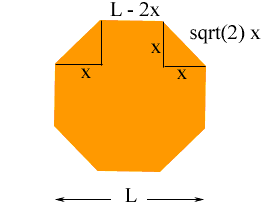Quandaries and Queries Hi; My name is Rich, If I know the distance between the parallel lines of an octagon and want to find the length of the sides, can I just divide that distance by 2.414 to reveal the length of the sides? I work construction and just curious if I am figuring it right.                                  Thanks for your help! Rich Hi Rich, I don't carry numbers like that around in my head, I have to work it out. I drew a regular octagon and called the distance between the parallel sides L. I drew right tringles in the corners and called the lengths of the sides of the triangle x.From the diagram you can see that the length of each side of the octagon is L - 2x. Also Pythagoras' theorem tells us that the length of the hypotenuse of the right triangle with sides of length x is sqrt(x2 + x2) = sqrt(2) x But this is the length of a side of the regular octagon and we know from above that the length of the sides are L - 2x. Thus L - 2x = sqrt(2) x and hence x = L/2 + sqrt(2) Thus the length of a side is sqrt(2) x = sqrt(2) L/2 + sqrt(2) = 1/2.414 L as you thought. Penny Go to Math Central